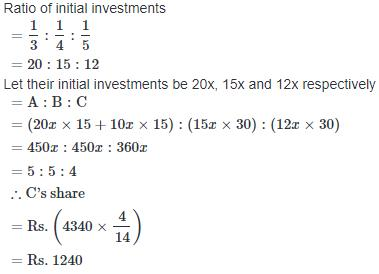Courses

# Test: Partnership 4

## 20 Questions MCQ Test Quantitative Techniques for CLAT | Test: Partnership 4

Description
This mock test of Test: Partnership 4 for Quant helps you for every Quant entrance exam. This contains 20 Multiple Choice Questions for Quant Test: Partnership 4 (mcq) to study with solutions a complete question bank. The solved questions answers in this Test: Partnership 4 quiz give you a good mix of easy questions and tough questions. Quant students definitely take this Test: Partnership 4 exercise for a better result in the exam. You can find other Test: Partnership 4 extra questions, long questions & short questions for Quant on EduRev as well by searching above.
QUESTION: 1

### A, B and C enter into a partnership. A initially invests Rs. 25 lakhs and adds another Rs. 10 lakhs after one year. B initially invests Rs. 35 lakhs and withdraws Rs. 10 lakhs after 2 years and C invests Rs. 30 lakhs. In what ratio should the profits be divided at the end of 3 years ?

Solution:

A : B : C
= (25 lakhs × 1) + 35 lakhs × 2) : (35 lakhs × 2 + 25 lakhs × 1) : (30 lakhs × 3)
= 95 lakhs : 95 lakhs : 90 lakhs
= 19 : 19 : 18

QUESTION: 2

### Subhash starts a business by investing Rs. 25000. 6 months later Aditya joins him by investing Rs. 15000. After another 6 months Aditya invests an additional amount of Rs. 15000. At the end of 3 years they earn a profit of Rs. 247000. What is Aditya's share in the profit ?

Solution: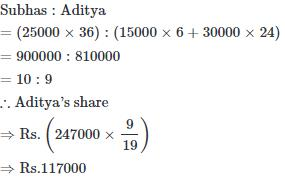QUESTION: 3

### A, B and C enter into a partnership with capitals in the ratio 5 : 6 : 8. At the end of the business term, they received the profit in the ratio 5 : 3 : 12. Find the ratio of time for which they contributed their capitals ?

Solution: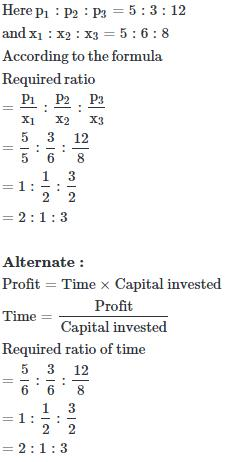QUESTION: 4

X and Z invest capital in the ratio of 2 : 1 while X and Y invest capital in the ratio of 3 : 2. If their annual profit is Rs. 157300 then what is Y share ?

Solution:

Given
X : Z = 2 : 1
X : Y = 3 : 2
X : Z = 2 : 1 (multiply with 3)
X : Y = 3 : 2 (multiply with 2)
i.e X : Z = 6 : 3
X : Y = 6 : 4

∴ X : Y : Z = 6 : 4 : 3
According to the question
(6+4+3)units = Rs.157300
13 units = Rs.175300
1 unit = Rs.12100
4 units = Rs.12100×4 = Rs. 48400
∴Share of Y = Rs. 48400

QUESTION: 5

A and B start a business with investments of Rs. 5000 and Rs. 4500 respectively. After 4 months, A takes out half of his capital. After two more months B takes out one-third of his capital while C joins them with a capital of Rs. 7000. At the end of a year, they earn a profit of Rs. 5080. Find the share of each member in the profit ?

Solution: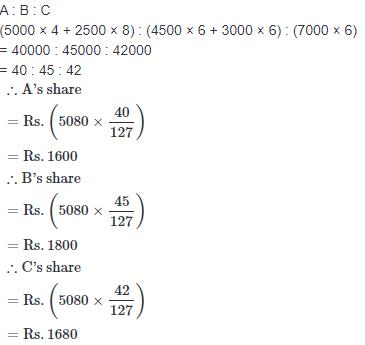QUESTION: 6

A, B and C subscribe Rs. 50000 for a business. A subscribes Rs. 4000 more than B and B Rs. 5000 more than C. Out of a total profit of Rs. 35000, A receives ?

Solution: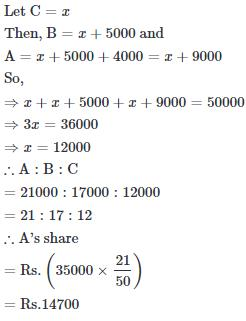QUESTION: 7

A, B and C are three partners. They altogether invested Rs. 14000 in business. At the end of the year, A got Rs. 337.50, B Rs. 1125 and C Rs. 637.50 as profit. The difference between the investments of B and A was ?

Solution: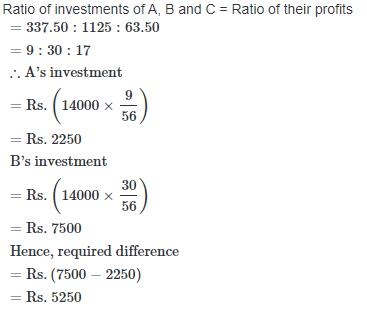QUESTION: 8

A and B are two partners in a firm sharing the profit in the ratio 4 : 5. If the firm earns a profit of Rs. 14130, then profit to be received by B ?

Solution: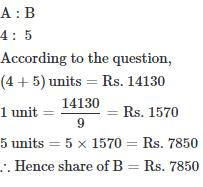QUESTION: 9

A and B started a business investing amounts in the ratio of 2 : 3. If A has invested an additional amount of Rs. 10000, their ratio of investment would have been 3 : 2. The amount invested by A was ?

Solution:

Initial ratio of investments by A and B = 2 : 3
Let their respective investments are 2x and 3x
According to the question,
If A added Rs. 10000 to his investment
Then the new ratio = 3 : 2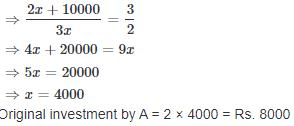QUESTION: 10

A, B and C started a business investing amounts in the ratio of 5 : 6 : 8 respectively. After one year, C withdrew 50% of the amount and A invested an additional amount of 60% of the original amount invested by him. In what ratio, the profit earned at the end of 2 years should be distributed among A, B and C respectively ?

Solution:

Let the initial investments of A, B and C be Rs. 5x, Rs. 6x and Rs. 8x respectively
Then, A : B : C
[5x × 12 + (160% of 5x)] : (6x × 24) : (8x × 12 + 4x × 12)
= 156x : 144x : 144x
= 13 : 12 : 12

QUESTION: 11

Jhon, Mona and Gordon, three US based business partners, jointly invested in a business project to supply nuclear fuel to India. As per their share in the investment, Gordon will receive 2/3 of the profits whereas Jhon and Mona divided the remainder equally. It is estimated that the income of Jhon will increase by \$ 60 million when the rate of profit rises from 4% to 7%. What is Mona's capital ?

Solution: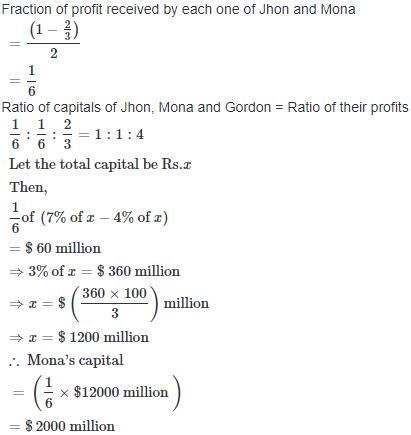QUESTION: 12

Anu is a working partner and Bimla is a sleeping partner in a business. Anu puts Rs. 5000 and Bimla putes in Rs. 6000. Anu receives 12.5% of the profit for managing the business and the rest is divided in proportion to their capitals. What does each get put of a profit of Rs. 880 ?

Solution: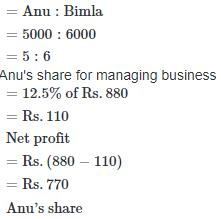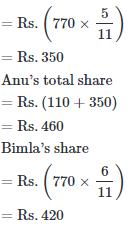QUESTION: 13

The ratio of investments of two partners X and Y is 11 : 12 and the ratio of their profit is 2 : 3. If X invested the money for 8 months, then the time for which Y invested the money is ?

Solution:

Let capital of X be Rs. 11x and Y's capital be Rs. 12x and let time for which Y invested capital is T2 months by using formula,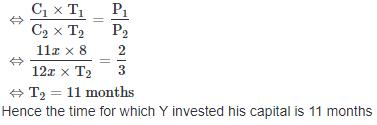QUESTION: 14

A and B enter into partnership. At the end of 9 months B withdraws but A's capitals is used for one month more. If they receive profit in the ratio of 5 : 6, then the ratio of their capital is ?

Solution: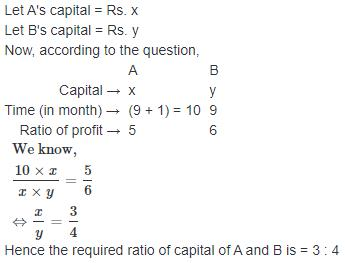QUESTION: 15

Two partners invested Rs. 125000 and Rs. 85000 respectively in a business. They distribute 60% of the profit equally and decide to distribute the remaining 40% as the interest on their capitals. If one partner received Rs. 3000 more than the other, the total profits is ?

Solution: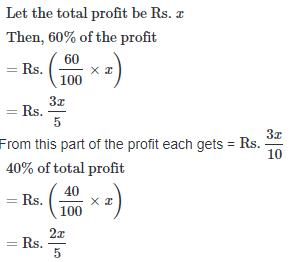Now, this amount of Rs. has been divided in the ratio of capitals, which is 125000 : 85000 or 25 : 17 as interests
∴ Interest on first capital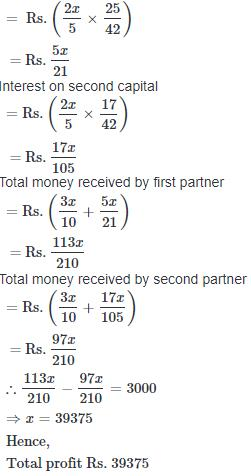QUESTION: 16

Nonad, Vikas and and Manav enter into a partnership. Ninad invest some amount at the beginning. Vikas invest double the amount after 6 months and Manav invests thrice the amount invested by Ninad after 8 months. They earn a profit of Rs. 45000 at the end of the year. What is Manav's share in the profit ?

Solution: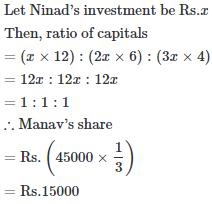QUESTION: 17

Four milkmen rented a pasture. A grazed 15 cows for 4 months, B grazed 12 cows for 2 months, C grazed 18 cows for 6 months and D grazed 16 cows for 5 months. If A's share of rent is Rs. 1020, what is C's share of rent ?

Solution: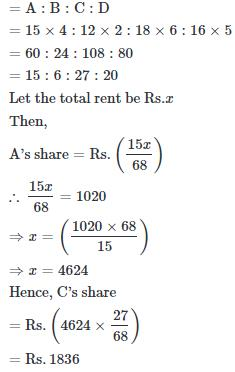QUESTION: 18

A, B and C are three partners in a business. The profit share of A is 3/16 of the total profit and B's share is of the total profit. If C receives Rs. 243, then the amount received by B will be ?

Solution: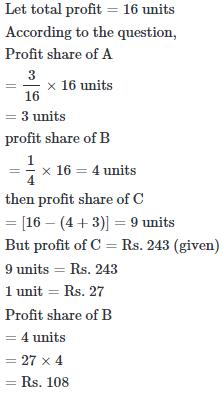QUESTION: 19

A is a active partner and B is a inactive partner in business. A puts in Rs. 5000 and B puts in Rs. 6000. A received 15% of the profit for managing the business and the rest is divided in proportion to their capitals. The amounts received by A out of the profit of Rs. 880 in all is ?

Solution: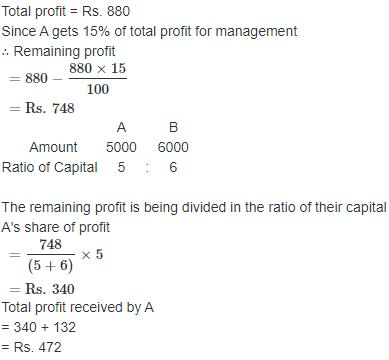QUESTION: 20

A, B and C are partners in a business. Their shares are in the proportion of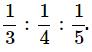A withdraws half of his capital after 15 months and after another 15 months, a profit of Rs. 4340 is divided. The share of C is ?

Solution: